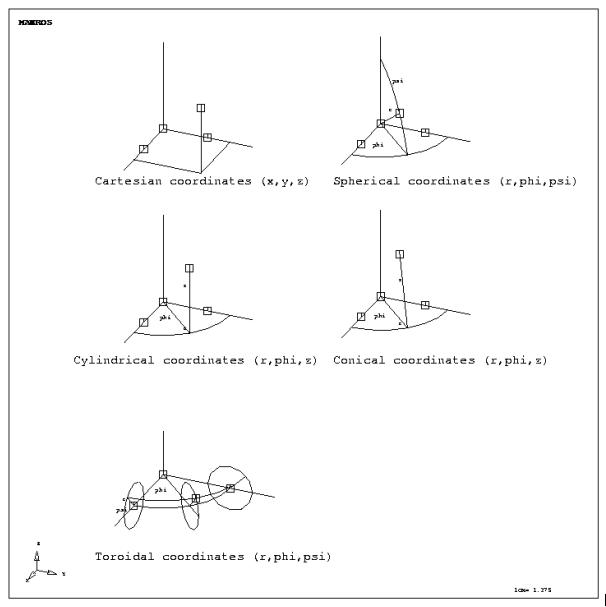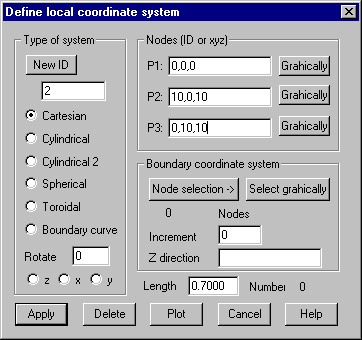Coordinate System: Define local coordinate system

Different coordinate systems can be defined to be used later by commands like Sort, Smooth, 3D-Extension/Translation, Boundary conditions, Nodal loads . These coordinate systems are saved together with the nodes of the macro and the FE model. In case a new structure is loaded from hard disk they currently defined coordinate systems are maintained if they are not overwritten by loaded systems with identical IDs. No longer needed coordinate systems have to be deleted explicitly.  The dialog shows the number of defined coordinate system.

The orientation of coordinate systems is defined by three nodes, which can be specified either by a node ID or by global coordinates (x,y,z).

Node P1 defines the origin, P2 defines the positive direction of the local x-axis and P3 defines a node somewhere within xy-plane (except for „cylindrical2“ systems). The local z-axis is always set up to build a right-handed cartesian coordinate system. Toroidal coordinate system uses P2 as the origin, i.e. the distance P1-P2 defines the torus radius.

Following figure shows available coordinate systems and the definition of these systems.Following dialog shows the available options:Type of system

ID: Give the ID of the coordinate system to be created or range of IDs for coordinate systems to be deleted, plotted or rotated. Button “New ID” gets the next not used ID.

Cartesian: Cartesian system with coordinates x,y,z

Cylindrical: Cylindrical system with coordinates r,j,z. Z-axis is perpendicular to plane P1-P2-P3

Cylindrical:2 Cylindrical system with coordinates r,j,z. Direction P1-P2 builds z-axis, P1-P3 build x-axis

Spherical: Spherical system with coordinates r,j,f. P1 = center node.

Toroidal: Toroidal system with coordinates r,j,f.

Boundary curve: This Option makes it possible to define multiple cartesian coordinate systems tangential to a given curve, for example for the definition of perpendicular or tangential loads or boundary conditions. The nodes of the curve must be selected within “Node selection“ dialog or graphically. For each node of the boundary curve the tangent vector is calculated. The x-axis of the coordinate system is oriented towards this vector and perpendicular to the given z-direction. The generated coordinate systems are numbered with the IDs of the related nodes plus the given increment. There are a maximum number of 500 coordinate systems that can be stored.

Rotate: This option makes it possible to alter the orientation of already defined local coordinate systems. The axis about which should be rotated must be selected and the angle of rotation must be given. The IDs of one or more defined coordinate systems can be given.

Nodes (ID or xyz):

Define points P1-P3 with node ID or global coordinates.

P1: The origin of the coordinate system.

P2: Node on x-axis.

P3: Node within xy-plane.

Graphically: The node is to be selected within the OpenGL window. The coordinates of these nodes will be updated within the corresponding input fields.

Boundary coordinate systems

When option “Boundary curve” is selected, the following additional parameters must be given:

Node selection: Node selection dialog pops up to define nodes for the boundary curve. The boundary curve is defined by all element nodes, which are contained within this selection.

Select graphically: Nodes of boundary curve will be selected graphically.

Increment: The increment for numbering the generated coordinate systems must be given (0 is allowed).

Z-direction: The direction of the z-axis of the local coordinate system must be given. This direction must not be within the plane of the boundary curve.

Delete

One or more coordinate systems are removed. The ID of the coordinate system or a range of IDs for several coordinate systems to be deleted must be given.

Plot

This button plots the coordinate systems who’s IDs are given. Also the length of the axis must be given. ID 0 plots all coordinate systems. Newly defined systems are automatically plotted.